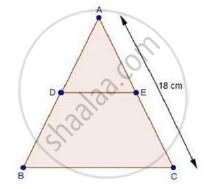Share

Books Shortlist
Your shortlist is empty

# Solution for In δAbc, D and E Are Points on the Sides Ab and Ac Respectively Such that De || Bc If "Ad"/"Db"=2/3 And Ac = 18 Cm, Find Ae - CBSE Class 10 - Mathematics

ConceptBasic Proportionality Theorem Or Thales Theorem

#### Question

In ΔABC, D and E are points on the sides AB and AC respectively such that DE || BC

If "AD"/"DB"=2/3 and AC = 18 cm, find AE

#### SolutionWe have,

"AD"/"DB"=2/3and DE || BC

Therefore, by basic proportionality theorem, we have

"AD"/"DB"="EC"/"AE"

rArr3/2="EC"/"AE"

Adding 1 on both sides, we get

rArr3/2+1="EC"/"AE"+1

rArr(3+2)/2=("EC"+"AE")/"AE"

rArr5/2="AC"/"AE"               [∵ AE + EC = AC]

rArr5/2=18/"AE"                 [∵ AC = 18]

rArr"AE=(18xx2)/5"

rArr"AE"=36/5=7.2 " cm"

Is there an error in this question or solution?

#### Video TutorialsVIEW ALL 

Solution for question: In δAbc, D and E Are Points on the Sides Ab and Ac Respectively Such that De || Bc If "Ad"/"Db"=2/3 And Ac = 18 Cm, Find Ae concept: Basic Proportionality Theorem Or Thales Theorem. For the course CBSE
S